2022: SklogWiki celebrates 15 years on-line

# Microcanonical ensemble

Microcanonical ensemble

## Ensemble variables

(One component system, 3-dimensional system, ... ):

•$\left. N \right.$: number of particles
•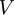$\left. V \right.$: is the volume
•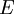$\left. E \right.$: is the internal energy (kinetic + potential)

## Partition function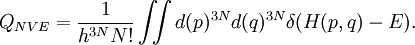$Q_{NVE} = \frac{1}{h^{3N} N!} \iint d (p)^{3N} d(q)^{3N} \delta ( H(p,q) - E).$

where:

•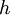$\left. h \right.$ is the Planck constant
•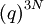$\left( q \right)^{3N}$ represents the 3N Cartesian position coordinates.
•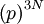$\left( p \right)^{3N}$ represents the 3N momenta.
•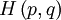$H \left(p,q\right)$ represents the Hamiltonian, i.e. the total energy of the system as a function of coordinates and momenta.
•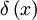$\delta \left( x \right)$ is the Dirac delta distribution

## Thermodynamics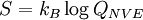$\left. S = k_B \log Q_{NVE} \right.$

where:

•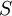$\left. S \right.$ is the entropy.
•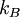$\left. k_B \right.$ is the Boltzmann constant